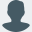# Generating correlated random numbers in R from scratchRiddhiman

Here’s a quick post on how to generate correlated random numbers in R inpired by this stack overflow post.

First step is to define a covariance matrix

``````# Covariance and correlation for standardised variables would be same
(cor_mat <- matrix(c(1, 0.3, 0.3, 1), nrow = 2, byrow = T))
##      [,1] [,2]
## [1,]  1.0  0.3
## [2,]  0.3  1.0
``````

Next decompose the matrix using Cholesky’s decomposition

``````(chol_mat <- chol(cor_mat))
##      [,1]      [,2]
## [1,]    1 0.3000000
## [2,]    0 0.9539392
``````

Generate some random numbers

``````old_random <- matrix(rnorm(2000), ncol = 2)
``````

Multiply this matrix with the upper triangular matrix from above

``````new_random <- old_random %*% chol_mat
cor(new_random)
##          [,1]     [,2]
## [1,] 1.000000 0.299686
## [2,] 0.299686 1.000000
``````
``````cor(old_random)
##           [,1]      [,2]
## [1,] 1.0000000 0.0106811
## [2,] 0.0106811 1.0000000
``````
``````cor(new_random)
##          [,1]     [,2]
## [1,] 1.000000 0.299686
## [2,] 0.299686 1.000000
``````

## Some notes and caveats

1. The original random variables need to be as uncorrelated as possible for this to work well.
``````corrs_high <- c()

for(i in 1:1000){

x <- rnorm(1000)
y <- 2 * x + rnorm(1000)

old_random <- as.matrix(data.frame(x, y))
chol_mat <- chol(matrix(c(1, 0.3, 0.3, 1), ncol = 2, byrow = T))
new_random <- old_random %*% chol_mat
corrs_high <- c(corrs_high, cor(new_random)[1,2])
}
``````
``````# The specified correlation/covariance structure is not respected
hist(corrs_high)
````````````corrs_low <- c()

for(i in 1:1000){

x <- rnorm(1000)
y <- 0.001 * x + rnorm(1000)

old_random <- as.matrix(data.frame(x, y))
chol_mat <- chol(matrix(c(1, 0.3, 0.3, 1), ncol = 2, byrow = T))
new_random <- old_random %*% chol_mat
corrs_low <- c(corrs_low, cor(new_random)[1,2])
}
``````
``````# Now the correlation between the two variables is much closer to the specified value
hist(corrs_low)
``````1. Tends to not work results if the original samples (uncorrelated random variables) are from different distributions
``````x <- rchisq(1000, 2, 3)
y <- rnorm(1000)

old_random <- as.matrix(data.frame(x, y))
chol_mat <- chol(matrix(c(1, 0.3, 0.3, 1), ncol = 2, byrow = T))
new_random <- old_random %*% chol_mat
``````
``````cor(new_random)
##           [,1]      [,2]
## [1,] 1.0000000 0.7868328
## [2,] 0.7868328 1.0000000
``````
``````x <- rchisq(1000, 2, 3)
y <- rchisq(1000, 2, 3)

old_random <- as.matrix(data.frame(x, y))
chol_mat <- chol(matrix(c(1, 0.3, 0.3, 1), ncol = 2, byrow = T))
new_random <- old_random %*% chol_mat
``````
``````cor(new_random)
##           [,1]      [,2]
## [1,] 1.0000000 0.2931943
## [2,] 0.2931943 1.0000000
``````
1. There is no way to ensure that characteristics of the original distributions are maintained
``````x <- rchisq(1000, 2, 3)
y <- rchisq(1000, 2, 3)

old_random <- as.matrix(data.frame(x, y))
chol_mat <- chol(matrix(c(1, -0.3, -0.3, 1), ncol = 2, byrow = T))
new_random <- old_random %*% chol_mat
``````
``````# While the correlation value seems fine
cor(new_random)
##            [,1]       [,2]
## [1,]  1.0000000 -0.3103062
## [2,] -0.3103062  1.0000000
``````
``````# There are negative values!
range(new_random)
##  -7.828389 28.882035
``````

Or, just use `mvtnorm::rmvnorm()` 😄

``````sigma <- matrix(c(4,2,2,3), ncol=2)
cov2cor(sigma)  ## Expected correlation
##           [,1]      [,2]
## [1,] 1.0000000 0.5773503
## [2,] 0.5773503 1.0000000
``````
``````x <- mvtnorm::rmvnorm(n = 500, mean = c(1,2), sigma = sigma)
cor(x)  ## Actual correlation
##           [,1]      [,2]
## [1,] 1.0000000 0.6057812
## [2,] 0.6057812 1.0000000
``````